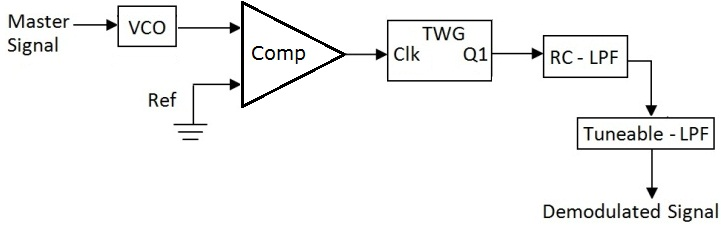FM - Demodulation

Theory

Slope Detector

The slope detector is a method of Frequency demodulation which converts the received FM signal to AM and demodulates with an envelope detector. Any circuit that outputs the time derivative of the input can perform the FM to AM conversion. In a FM signal the frequency is low, when the amplitude of the message signal is low and vice versa.Slope detection converts the FM signal to Amplitude modulated AM-FM signal using the slope of a filter circuit. Since the peak-to–peak amplitude of the FM signal is constant; on the up-slope of a band pass filter circuit is a lower frequency yield a lower voltage, a higher frequency will yield a higher voltage. When the output of the filter is envelope detected, the original message signal is recovered.Fig.1 Block diagram illustrating the FM-Demodulation.

The slope detection method revolves around a differentiation operation that exploits the instantaneous frequency of the FM signal. The FM input signal is first subjected to a limiter in order to eliminate any amplitude modulation noise present in the signal. The output of the limiter is a square wave with constant amplitude. The square wave is then sent through the band pass filter. The BPF filter out the square wave harmonics and returns a constant-amplitude sinusoid. The constant amplitude FM signal is then differentiated. The differentiation of the cosine carrier function exploits the instantaneous frequency of the FM signal by the property of the chain rule. Now the instantaneous frequency can be thought of as the time varying amplitude of the cosine carrier function originates. The last step is to subject the differentiated FM signal to an envelope detector. The envelope detector extracts the amplitude, or envelope of the input signal of interest. In the slope detection case, the extracted envelope is the instantaneous frequency of the FM signal, which contains the original message m (t). An ideal frequency modulation detector is a device that produces an output that is proportional to the instantaneous frequency of the input. Slope detection is a type of FM to AM conversion.

To recover the information contained in a FM signal requires obtaining the signals instantaneous frequency.The instantaneous radian frequency is

ωi = ωc + Kf m (t)

Where Kf = 2fd

A network which has a magnitude response of the form | H(t) | = a ( f - fc ) + b, in the vicinity of the carrier frequency fc, will convert into an AM type signal with an envelope proportional to the instantaneous frequency. Envelope detection can then be used to obtain m(t). Mathematically the easiest example is the ideal differentiator which has transfer function j2f.

The differentiator output isWhere the envelope Ac [ ωc + Kf m(t) ] is clearly proportional to m(t) since ωc is fixed.

Note: The RC LPF and Tuneable LPF modules are used in combination because the Tuneable LPF is a clocked "switched capacitor" filter which uses internal sampling technology. These types of filters are subject to aliasing which can cause problems in sampled systems. To avoid such problems, we use the RC LPF as an anti-aliasing pre-filter for the Tuneable LPF. If time permits, explore this part of the experiment without the RC LPF.